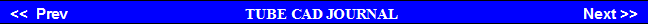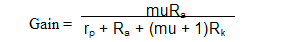The first step is to find the intersecting points on the gridlines where the corresponding current equals the current draw from the cathode resistor at the gridline's voltage (in absolute terms). This sounds complicated, but isn't. For example, in the graph above we see the plate resistor's line plotted from one corner to the other and we see the cathode resistor's line stretching from the intersection with the -2 and -6 gridlines. At these two points (20 mA and 60 mA) the 100-ohm resistor will find 2 and 6 volts across its leads.    Now the intersection of the cathode resistor and plate resistor lines should give us the idle current and the plate voltage -- but it doesn't. We face the same task we did before of having to shift the plate curves to the right to compensate for the voltage lost across the cathode resistor. But for most situations, the intersection is close enough.Optimal Plate Resistor Values    We know how to determine the values of the plate resistor and the cathode resistor, but which values are best? This question leads to another question: Best for what? Do we need the greatest amount of gain or the greatest voltage swing? Producing the greatest gain requires using the largest plate resistor possible and bypassing the
 cathode resistor with a large valued capacitor, as the following formula reveals:Gain =   muRa  / (rp + Ra) for bypassed cathode resistors andfor unbypassed cathode resistors. There are limits, however. Unless we are willing to use an extremely high power supply--say, 1 kV--we must accept a mere trickle current to accommodate a plate resistor that equaled 20Rk.     The danger of such a light idle current lies in the dragging down of the high frequency response due to the circuit's inability to charge the load (and stray) capacitance. Besides, the triode is least linear at the bottom of its conduction and the greatest amount of gain is not the same as the greatest symmetrical voltage swing.      (The hidden advantage to trickle currents is that the power supply design is greatly relived by wimpy idle currents, as the power transformer and DC filtering capacitor can be made smaller, i.e. cheaper; furthermore, it greatly extends tube life. But light current is not compatible with linearity and, over time, can lead to problems like the dreaded sleeping sickness. So, as many will agree, it is better to burn-out than rust away. )www.tubecad.com   Copyright © 2003 GlassWare   All Rights

Pg.

10